Reach Us+44-1235-425476
Application of a Modified Homotopy Perturbation Method for Calculation of Secular Axial Frequencies in a Nonlinear Ion Trap with Hexapole, Octopole and Decapole Superpositions
Journal of Bioanalysis & Biomedicine

Like us on:

All submissions of the EM system will be redirected to Online Manuscript Submission System. Authors are requested to submit articles directly to Online Manuscript Submission System of respective journal.

# Application of a Modified Homotopy Perturbation Method for Calculation of Secular Axial Frequencies in a Nonlinear Ion Trap with Hexapole, Octopole and Decapole Superpositions

Alireza Doroudi*

Physics Department, Nuclear Science Research School, Nuclear Science and Technology Research Institute (NSTRI), P.O. Box 14395-836, Tehran, Iran

*Corresponding Author:
Amineh Rezaeian Asl
Department of Physics
Faculty of Science
Islamic Azad University
Central Tehran Branch
Tehran, Iran
E-mail: [email protected]

Received Date: September 09, 2012; Accepted Date: October 17, 2012; Published Date: October 22, 2012

Citation:Doroudi A (2012) Application of a Modified Homotopy Perturbation Method for Calculation of Secular Axial Frequencies in a Nonlinear Ion Trap with Hexapole, Octopole and Decapole Superpositions. J Bioanal Biomed 4:085-091. doi:10.4172/1948-593X.1000068

Copyright: © 2012 Doroudi A. This is an open-access article distributed under the terms of the Creative Commons Attribution License, which permits unrestricted use, distribution, and reproduction in any medium, provided the original author and source are credited.

Visit for more related articles at Journal of Bioanalysis & Biomedicine

#### Abstract

In this paper we have used a modified homotopy perturbation method used previously by A. Belendez and his
coworkers, for calculation of axial secular frequencies of a nonlinear ion trap with hexapole, octopole and decapole superpositions. We transform the motion of the ion in a rapidly oscillating field to the motion in an effective potential and obtain a nonlinear differential equation in the form of a Duffing-like equation. With only octopole superposition the resulted nonlinear equations are symmetric; however, in the presence of hexapole and decapole superpositions, they are asymmetric. For asymmetric oscillators, it has been pointed out that the angular frequency for positive amplitudes is different from the angular frequency for negative amplitudes. Considering this problem, the modified homotopy perturbation method is used for solving the resulted nonlinear equations. As a result, the ion axial secular frequencies as a function of nonlinear field parameters are obtained. The calculated secular frequencies are compared with the results of modified Lindstedt-Poincare approximation and the exact results. There is an excellent agreement between the results of this paper and the exact results.

#### Keywords

Modified homotopy perturbation; Nonlinear ion trap; Secular frequency; Hexapole; Octopole; Decapole; Symmetric oscillator; Asymmetric oscillator

#### Introduction

In an ideal ion trap the potential is pure quadrupole and the main properties of the movement of an ion are obtained by the solution of Mathieu equation . In a practical ion trap, however, the electric field distribution deviates from linearity which is the characteristic of pure quadrupolar trap geometry. This deviation is caused by many different agents such as the truncation of electrodes.

The nonlinearity in ion traps superimpose weak multipole fields (e.g., hexapole, octopole, decapole, and higher order fields) and the resulting nonlinear field ion traps exhibit some effects which differ considerably from those of the linear field traps.

The equation governing the motion of the ion in the nonlinear ion trap is the nonlinear Mathieu equation which cannot be solved analytically. The superposition of weak higher multipole fields changes the motions of ions compared to their motions in a pure quadrupole ion trap.

Simulation studies  have shown that hexapole superposition decreases the secular frequency, positive octopole superposition increases the ion secular frequency and the negative octopole superposition decreases the secular frequency. Experimentally, it has been shown that  the octopole and hexapole superposition resulted in a decrease in ion secular frequency.

Sevugarajan and Menon [4-6] have studied the nonlinear Paul ion trap. They have applied the Lindstedt-Poincare technique, the modified Lindstedt-Poincare technique and the multiple scales perturbation technique for solving the nonlinear equation of ion motion in nonlinear ion trap. Also, in two previous studies [7,8] done on nonlinear ion traps by one of the present authors, the homotopy perturbation method was used to study the secular frequencies in nonlinear ion traps. When the hexapole superposition is considered, the resulting nonlinear equation has a quadratic nonlinearity and we know that the angular frequency for positive amplitudes is different from the angular frequency for negative amplitudes in nonlinear oscillator with quadratic nonlinearity. In all the above studies [4-8] the assumption is that the angular frequency for positive amplitudes is equal to the angular frequency for negative amplitudes.

In studying the quadratic nonlinear oscillator and mixed parity nonlinear oscillator by the method of harmonic balance, H. Hu [9,10] has used the sign function for incorporating the inequality of angular frequency for negative amplitudes and positive amplitudes. In a recent study , this technique was used in the framework of the parameter expanding or modified Lindstedt-Poincare method [12-14] for calculation of secular axial frequencies in a nonlinear ion trap with hexapole, octopole and decapole superpositions. In this paper, we use the same technique and the homotopy perturbation method [14-25] with a modification [26-28] for studying the asymmetric nonlinear oscillators. The modification in He’s homotopy perturbation method is introduced by truncating the infinite series corresponding to the first order approximate solution before introducing this solution in the second order linear differential equation and so on.

The exact solution for nonlinear equation of an anharmonic oscillator with quadratic nonlinearity and the exact expression for its period have been studied by some authors [29,30]. They have found the exact expression for the period of nonlinear oscillator in terms of complete elliptic integrals. We have used the results of these papers and have calculated the exact frequencies of an anharmonic oscillator with quadratic nonlinearity. The mathematica software has been used for calculation of elliptic integrals.

In this paper we take into account the first four multipole terms of potential distribution inside the ion trap, i.e. quadrapole, hexapole, octopole and decapole terms and ignore the higher multipole fields. The resulting nonlinear equation has quadratic and cubic as well as quartic nonlinearity. Due to the inequality of angular frequency for negative amplitudes and positive amplitudes, we use the sign function for constructing the two auxiliary nonlinear equations. Then, the modified homotopy perturbation method [26-28] is used for solving the two auxiliary nonlinear differential equations and the ion secular frequencies are calculated. We compare the results of this paper with those obtained by using the modified Lindstedt-Poincare method  and with the exact results.

The outline of the paper is as follows: In equation 2 the axial equation of ion motion in a nonlinear ion trap is derived. In equation 3 the modified homotopy perturbation method is applied to solve the equation of ion motion in nonlinear ion trap and the results are also given in this equation. Finally, the concluding remarks are given in equation 4.

#### The Axial Equation of Ion Motion in a Nonlinear Ion Trap

The axial equation of ion motion in the presence of hexapole and octopole superpositions has been derived in [7,8] and in the presence of hexapole, octopole, and decapole superposition has been derived in . However, in this equation, we give a brief derivation of the axial equation of ion motion in the latter case.

A solution of Laplace’s equation in spherical polar coordinates for a system with axial symmetry can be written in the following general form :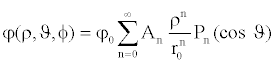(1)

where φ0 =U +V cosΩt is the potential applied to the trap, An’s are arbitrary dimensionless coefficients, Pn (cosϑ) denotes a Legendre polynomial of order n, and r0 is a scaling factor (i.e., the internal radius of the ring electrode).

When Pnρn(cosϑ ) is expressed in cylindrical polar coordinates (r, z) and the three higher order multipoles, i.e. hexapole, octopole and decapole corresponding to n = 3 , 4 and 5 along with the quadrupole component corresponding to n = 2 are taken into account, the time dependent potential distribution inside the trap takes the form: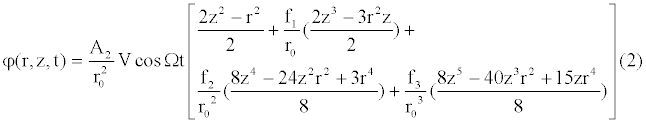where f1 = A3/ A2 , f2 = A4/ A2 and f3 = A5/ A2 . Here we have assumed the operation of the trap along the au = 0 axis in the Mathieu stability plot, that is, the DC component of ?0 is equal to zero. The coefficients A2 , A3 , A4 and A5 refer to the weight of the quadrupole, hexapole, octopole and decapole superpositions, respectively.

According to classical mechanics , the motion of an ion in a rapidly oscillating field such as ? (r, z,t) (due to the largeness of Ω) can be averaged and transformed to the motion in an effective potential, Ueff (r, z), related to ? (r, z,t) through the following relation: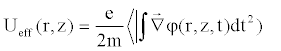(3)

Insertion of Eq. (2) for ? (r, z,t) into Equation. (3) and averaging with respect to time gives the following relation for Ueff (r, z),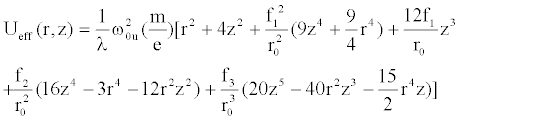(4)

where λ = 2 for u = r (radial direction) and λ = 8 for u = z (axial direction).

By ignoring the term proportional to f12 compared with the term proportional to f1 (because f1= A3/ A2 is small in comparison to 1), the final form of Ueff (r, z) reduces to the following form,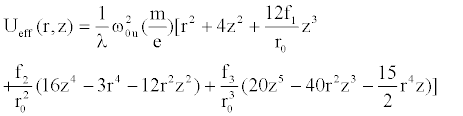(5)

The classical equation of ion motion in the effective potential Ueff (r, z) , and with no excitation potential applied to the end cap electrodes is given by: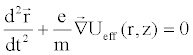(6)

Where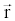is the position vector of the ion. Combining equations (5) and (6), we get the equation of motion in the axial (z) direction as: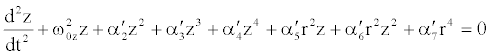(7)

This is the equation in z direction which is coupled to equation in r direction. Since we are interested in axial secular frequencies, we put r = 0 in equation (7) and get an equation in axial direction which depends only on z variable: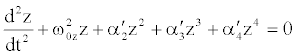(8)

where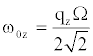(9)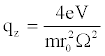(10)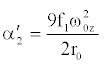(11)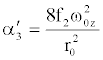(12)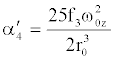(13)

In Equation (8), by introducing the dimensionless variable x through the relation x = z/ r0 , and omission of index z from ω0z (for simplicity) we get the equation,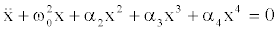(14)

where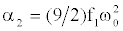,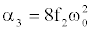and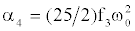There are several methods [33,34] that can be used for solution of the nonlinear Equation (14). In the next equation of this article we have used the modified homotopy perturbation method for solving this equation.

#### Application of Modified Homotopy Perturbation Method for Solution of the Axial Equation of Motion and the Results

The homotopy perturbation method [14-25] is a general method for solving the differential and integral equations. This method is explained in references 7 and 8 for solving a nonlinear differential equation like Equation (14). In this method an embedding parameter p∈[0,1] is introduced. The periodic solution of the nonlinear differential equation and the coefficient of linear term ( ω02 ) are assumed to be written as power series in p. Then, the power series for the solution and ω02 are inserted in the nonlinear equation and a set of linear differential equations is obtained for the coefficients of the power series for the periodic solution. The first few linear equations of the set are solved and then are inserted in the power series along with p =1 . In this manner, an approximate solution of the main nonlinear differential equation is obtained. Here we use this method for solving the equation (14).

The nonlinear differential equationis the equation of a mixed parity nonlinear oscillator and the amplitudes of oscillations for this oscillator are not the same when x ≥ 0 and x ≤ 0 . We assume that the positive amplitude is A and the negative amplitude is −B ( B is positive). Now we construct the two auxiliary equations by using sign function: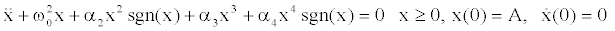(15)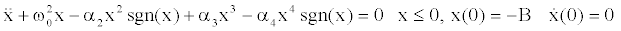(16)

The sign function is defined as: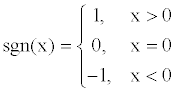(17)

First, we consider the Equation (15) and construct the following homotopy: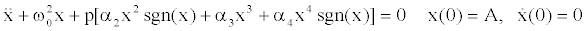(18)

In homotopy perturbation method the solution x and the constant ω02 are expanded in powers of embedding parameter p as: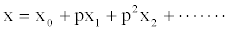(19)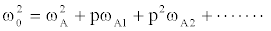(20)

In equation (15), the amplitude is A , so we have used the index A in the expansion of ω02 . As a result, in the expansion of ω02 for solution of equation (16) we use the index B.

Substitution of the power series (19) and (20) into Equation (18), and collecting terms of the same power of p, gives the following set of linear equations: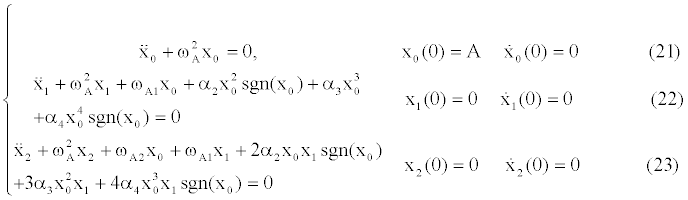In Equation (21)-(23) we have taken into account the following expression [35,36],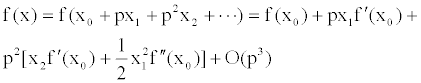(24)

where f(x) = df (x) / dx and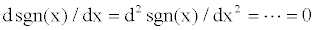for x ≠ 0 and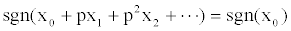The first equation of the set can be solved easily, giving the solution x0(t) = A cosωAt we can expand the terms x20sgn( x0) and x40sgn(x0) in Equation (22) in Fourier series as: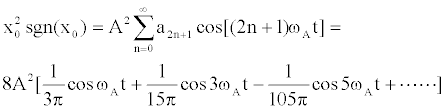(25)

Where,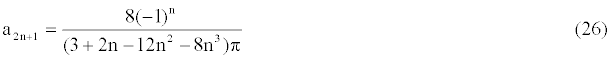and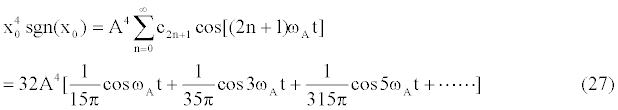where,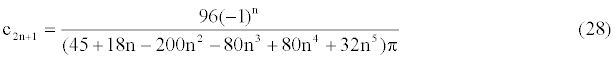Substituting x0(t) , as well as the above series expansions for x20sgn( x0) and x40sgn(x0) into Eq. (22), having no secular term in solution x1(t) requires eliminating contributions proportional to cosωAt in Eq. (22) and we obtain: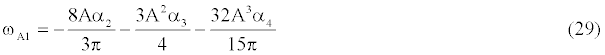Insertion of ωA1 into Eq. (20), neglecting the terms proportional to p2 and higher degrees, and combining with p =1 at last step, the approximate amplitude dependent frequency, ωA(1) , in first order is obtained as: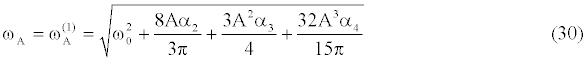Finally, insertion of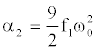,and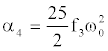in this equation gives the result,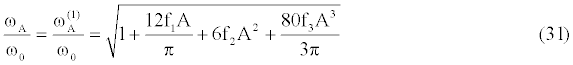In a similar way, for oscillation of ion in negative direction, from Eq. (16) we get the following result for ωB , in first order of perturbation,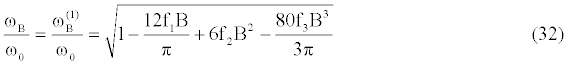In first order of the perturbation, the results for ωA and ωB are the same as the results of modified Lindstedt-Poincare method .

Now, we go to second order approximation. In order to go to second order approximation we need to obtain the correction term x1(t) for the periodic solution x0(t) . In order to obtain x1(t) , we insert the expression for ωA1 from Eq. (29) into Eq. (22) and rewrite it in the following form,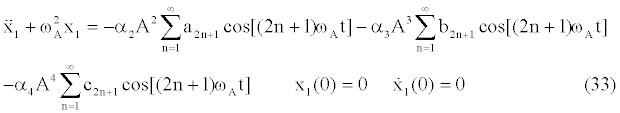where,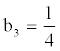and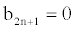for n ≥ 2.

Now, the periodic solution to Equation (33) can be written in the following form,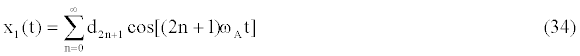Insertion of Equation (34) into Equation (33) gives the following expression for the coefficients d2n+1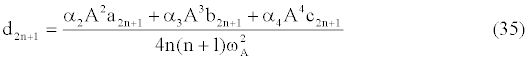for n ≥1.

Taking into account the initial condition x1(0) = 0 , we obtain from Equation (34):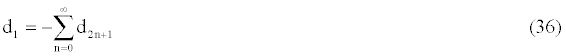To determine the second order approximate solution we need to substitute Equation (34) into Equation (23). The new differential equation for x2(t) is difficult to solve because of an infinite number of harmonic terms in x1(t). At this stage we consider the modification in He’s homotopy perturbation method [26-28] to simplify the solution procedure. In this modified version of homotopy method the infinite series expansion for x1(t)in Equation (34) is truncated and an approximate equation x1(N )(t) is written as: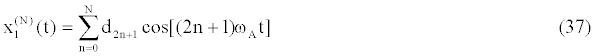where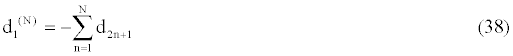In the approximate expression for x1(N )(t) we assume N = 2 and obtain the approximate solution for x1 as: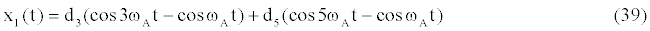d3 and d5 are calculated by using the relation (35).

Now, we substitute x0(t) and the approximate x1(t) into Equation (23) and expand the terms 2x0x1 sgn(x0) and 4x03x1sgn(x0) of this equation in Fourier series. Having no secular term in solution x2(t) requires eliminating contributions proportional to cosωAt in the resulted differential equation for x2(t), which implies: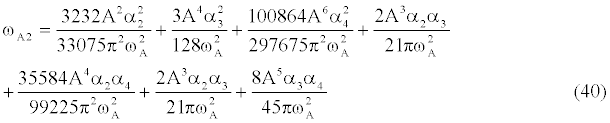Insertion of calculated expressions for ωA1 and ωA2 into Eq. (20) along with p =1 and using the values of,andgives the final result for ωA(2) , the second order approximate frequency and TA2 , the second order approximate period, in positive direction: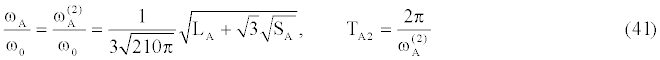Where,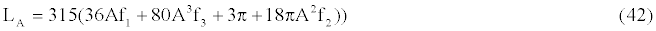and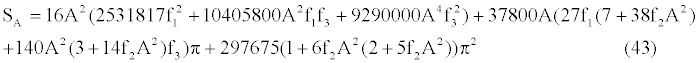In a similar way, for oscillation of ion in negative direction we get from Equation (16):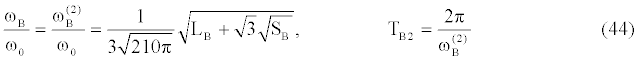Where,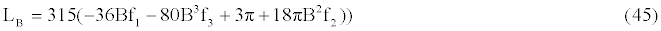and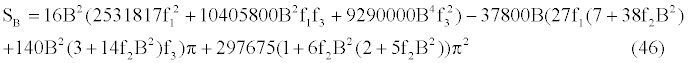In the relations (42) and (43) A is the positive amplitude and is equal to maximum value for x and xmax can be obtained by using the relation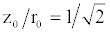for ion trap and inserting z0 for z in equation x = z0/ r0 gives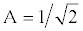. As mentioned earlier, the amplitude in negative direction, B , is different from A and can be calculated in terms of A . For calculation of B , the both side of Equation (14) is multiplied by x? and then integrated , giving the result,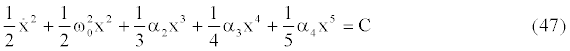Where C is the constant of integration. Insertion of initial conditions in Equation (47) gives the result,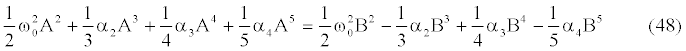Using mathematica software, this equation can be solved analytically and B is calculated in terms of A .

It is clear that the second order approximate period is: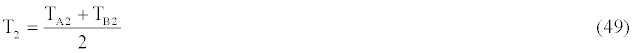So, the second order approximate secular frequency is: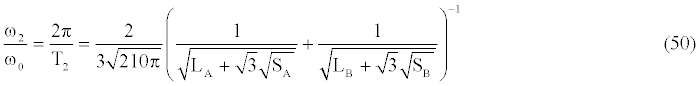The perturbed secular frequencies can be calculated through the relation (50) as a function of field aberrations (parameters f1 , f2 and f3)

The values of ω/ ω0 for different values of f1 , f2 and f3 are given in tables 1,2,3 and 4 and for comparison purposes the values of ω/ ω0 in modified Lindstedt-Poincare approximation  are also given in the tables.

f1 B ω2MHP/ ω0 ω2MLP /ω0 ωexact/ ω0
0.01
0.05
0.10
0.11
0.12
0.13
0.14
0.15
0.155
0.1565
0.722439
0.792221
0.913755
0.947125
0.986189
1.03385
1.09633
1.19283
1.28435
1.3404
0.999569
0.987927
0.939194
0.921209
0.898183
0.867497
0.8232207
0.744627
0.649963
0.556534
0.999568
0.987913
0.939082
0.921043
0.897928
0.867083
0.822454
0.742729
0.644365
0.527906
0.999569
0.987926
0.939193
0.921214
0.898204
0.867561
0.823418
0.745686
0.655595
0.582921

Table 1: Comparison of the calculated values of ω20 in this paper for only hexapole superposition with the values obtained by modified Lindstedt-Poincare approximation  and the exact values.

B ω2MHP/ ω0 ω2MLP /ω0 ωexact/ ω0
0.01
0.05
0.10
0.15
0.20
0.25
0.30
0.40
0.50
-0.01
-0.05
-0.10
-0.15
-0.20
1.01487
1.072
1.13891
1.20173
1.2612
1.31776
1.37188
1.4739
1.56905
0.984866
0.921355
0.833427
0.730894
0.598426
1.01487
1.072
1.1389
1.20173
1.2612
1.31776
1.37188
1.4739
1.56905
0.984866
0.921355
0.833427
0.730894
0.598426
1.01487
1.072
1.1389
1.2017
1.2612
1.3177
1.372
1.4739
1.569
0.98487
0.92136
0.83343
0.73099
0.59968

Table 2: Comparison of the calculated values of ω20 in this paper for only octopole superposition with the values obtained by modified Lindstedt-Poincare approximation  and the exact values.

f1 f1 B ω2MHP/ ω0 ω2MLP /ω0 ωexact/ ω0
0.01
0.05
0.10
0.15
0.20
0.25
0.12
0.05
0.07
0.01
0.01
0.05
0.10
0.15
0.20
0.25
0.30
0.05 -
0.07 -
0.10 -
0.721825
0.775049
0.829421
0.872078
0.905442
0.931747
0.794033
0.824202
0.979204
0.733768
1.01478
1.07113
1.13922
1.20686
1.27406
1.34028
1.39885
0.883214
0.698859
0.82468
1.01478
1.07113
1.13924
1.20693
1.27422
1.34054
1.39889
0.883152
0.697807
0.824691
1.01478
1.07113
1.13922
1.20689
1.27412
1.3404
1.39888
0.883223
0.700873
0.824691

Table 3: Comparison of the calculated values of ω20 in this paper for hexapole and octopole superpositions with the values obtained by modified Lindstedt-Poincare approximation  and the exact values.

 f1 f1 f1 B ω2MHP/ ω0 ω2MLP /ω0 ωexact/ ω0 0.01 0.02 0.10 0.05 0.07 0.15 0.12 0.14 0.13 0.03 0.05 0.01 0.02 0.01 0.02 0.10 0.05 0.08 0.20 0.15 0.30 0.40 -0.05 -0.01 0.10 - -0.08 0.01 0.02 0.06 0.03 0.05 0.10 0.04 0.12 0.16 0.02 0.04 0.01 0.03 0.73513 0.76452 0.966336 0.823497 0.885144 1.07527 0.894785 0.949597 0.92592 0.825353 0.935453 0.761675 0.89998 1.01302 1.02191 1.03587 1.0494 1.06025 1.08029 1.17802 1.31772 1.43909 0.869297 0.844398 0.808686 0.68875 1.01302 1.02192 1.03603 1.04941 1.0603 1.0811 1.178 1.31768 1.43654 0.869339 0.844862 0.808704 0.702734 1.01302 1.02191 1.03584 1.0494 1.06025 1.08014 1.17802 1.31774 1.43667 0.869302 0.844401 0.808711 0.69135

Table 4: Comparison of the calculated values of ω20in this paper for hexapole, octopole and decapole superpositions with the values obtained by modified Lindstedt- Poincare approximation  and the exact values.

For a nonlinear oscillator with only a quadratic term as a nonlinearity (α2 ≠ 0 and α3 =α4 = 0) , the exact values of frequencies are available in the literature [29,30] and are given in terms of complete elliptic integrals (relation No. (46) of ). Mathematica software has been used for calculation of numerical values of elliptic integrals and finding the roots of cubic polynomial equations. For a nonlinear oscillator with mixed parity (α2 ≠ 0 , α3 ≠ 0, α4 ≠ 0) , the exact values of frequencies can be calculated  by the integral,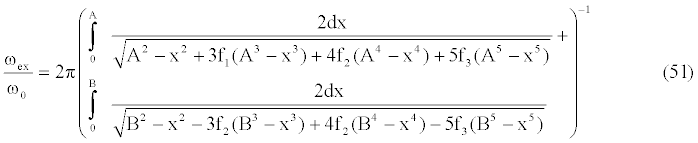This integral is evaluated numerically by mathematica for ωexact/ ω0 , the exact values of frequencies.

In Table 1, it has been assumed that only the hexapole superposition exist and the other multipoles are absent. So, the exact values of secular frequencies (ωexact/ ω0) for different values of f1 ( f2 = f3 = 0)are compared with the results of this paper for second order approximation (ω2MHP/ ω0) and the results of modified Lindstedt- Poincare approximation (ω2MLP /ω0) obtained in .

In Table 2, we have considered only the octopole superposition. In this table, the exact values of secular frequencies (ωexact/ ω0 ) for different values of f2 ( f1 = f3 = 0 ) are compared with the results of this paper for second order approximation (ω2MHP/ ω0) and the results of modified Lindstedt-Poincare approximation (ω2MLP /ω0) obtained in .

In Table 3, the hexapole and octopole superpositions are considered and the exact values of secular frequencies (ωexact/ ω0) for different values of f1 and f2 ( f3 = 0 ) are compared with the results of this paper for second order approximation (ω2MHP/ ω0) and the results of modified Lindstedt-Poincare approximation (ω2MLP /ω0) obtained in .

Finally, in Table 4, the hexapole, octopole, and decapole superpositions are considered and the exact values of secular frequencies (ωexact/ ω0) for different values of f1 , f2 and f3 are compared with the results of this paper for second order approximation (ω2MHP/ ω0) and the results of modified Lindstedt-Poincare approximation (ω2MLP /ω0) obtained in .

As is seen in the Table 1-4, the results of this paper are in excellent agreement with the exact results and are closer to the exact results compared with the results of modified Lindstedt-Poincare approximation obtained in .

#### Conclusion

In this paper we have derived the equation of ion motion in axial direction of a nonlinear ion trap. The nonlinear ion trap is generated by superposition of weak multipole fields on the pure quadrupole field. Hexapole, octopole, and decapole field superpositions are considered. The computed axial equation of ion motion is a nonlinear equation with quadratic, cubic and quartic nonlinearity. We have used the modified homotopy perturbation method for solution of the resulted equation and calculation of the axial secular frequencies of the ions in the trap. The results of this paper are compared with the exact results and the results of the modified Lindstedt-Poincare approximation obtained in . There is an excellent agreement between the results of this paper and the exact results.

#### References

Select your language of interest to view the total content in your interested language

### Article Usage

• Total views: 12263
• [From(publication date):
October-2012 - Aug 24, 2019]
• Breakdown by view type
• HTML page views : 8430
• PDF downloads : 3833

## Post your commentCan't read the image? click here to refresh
###### Peer Reviewed Journals

Make the best use of Scientific Research and information from our 700 + peer reviewed, Open Access Journals

International Conferences 2019-20

Meet Inspiring Speakers and Experts at our 3000+ Global Annual Meetings

Top